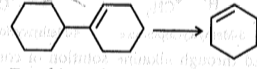To achieve above conversion, the reagents used will be:

(a) O3/H2O2, HO-/$∆$                                 (b) HBr, alc. KOH, O3, LiAlH4, H+/$∆$

(c)  HBr, t-BuOK, O3, KMnO4$∆$

High Yielding Test Series + Question Bank - NEET 2020

Difficulty Level: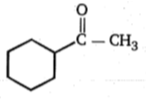; Product (B) is: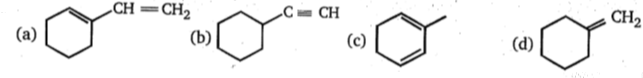High Yielding Test Series + Question Bank - NEET 2020

Difficulty Level:

CH3CH2C$\equiv$CH$\underset{\mathrm{liq}.{\mathrm{NH}}_{3}}{\overset{{\mathrm{NaNH}}_{2}}{\to }}$I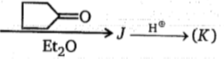Product (K) of the above reaction is:

(a)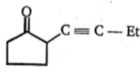(b)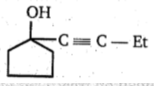(c)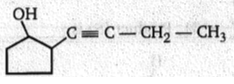(d)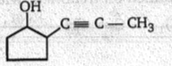High Yielding Test Series + Question Bank - NEET 2020

Difficulty Level:

CH3-CH2-CH2-C$\equiv$CH+LiHN2$\to \left(\mathrm{A}\right)\stackrel{\left({\mathrm{CH}}_{3}{\right)}_{2}{\mathrm{SO}}_{4}}{\to }$(B)

Give the structural formula of compound (B):

(a) CH3-(CH2)2-C$\equiv$C-SO3H

(b) CH3-(CH2)2-C$\equiv$C-CH3

(c)  CH3-(CH2)2-C$\equiv$C-CH2-O-$\begin{array}{c}\stackrel{\mathrm{O}}{\parallel }\\ \mathrm{s}\\ \underset{\mathrm{O}}{\parallel }\end{array}$-H

(d) CH3-CH2-C$\equiv$C-CH2

High Yielding Test Series + Question Bank - NEET 2020

Difficulty Level: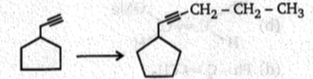; This conversion can be achieved by: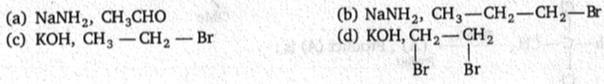High Yielding Test Series + Question Bank - NEET 2020

Difficulty Level:

Which alkyne will give 3-ethylhexane on catalytic hydrogenation?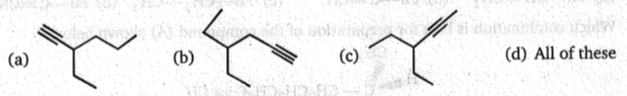High Yielding Test Series + Question Bank - NEET 2020

Difficulty Level:

Lindlar
Br-(CH2)12-C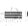CH
(A)(B);Product (B) is :
NaNH2   Catalyst
1.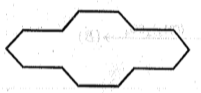2.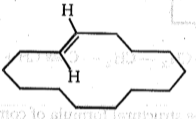3.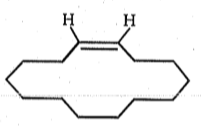4.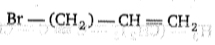High Yielding Test Series + Question Bank - NEET 2020

Difficulty Level:

Ph - C $\equiv \mathrm{CH}\underset{\mathrm{MeOH}}{\overset{{\mathrm{MeO}}^{-}}{\to }}$ Major product of the reaction is:

(a)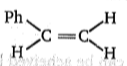(b)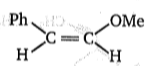(c) Ph - C $\equiv$C-OMe

(d)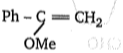High Yielding Test Series + Question Bank - NEET 2020

Difficulty Level:

; Product (A) is:

(a) Ph-CH=CH2          (b) Ph-C$\equiv$CH              (c) Ph-CH2-CH3             (d) Ph-C$\equiv$$\stackrel{⊝}{\mathrm{C}}\stackrel{\oplus }{\mathrm{Na}}$

High Yielding Test Series + Question Bank - NEET 2020

Difficulty Level: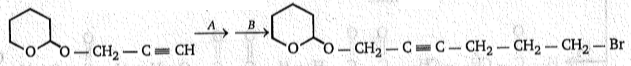To carry out above conversion, (A) and (B) respectively, are :

(a) NaNH2, Cl - CH2ーCH- CH- Br
(b) NaNH2, CH2-CH2-CH2-Br
(c) NaNH2, l-CH2ーCH2-CH2-Br
(d) NaNH2, I-CH2-CH2-CH2-I